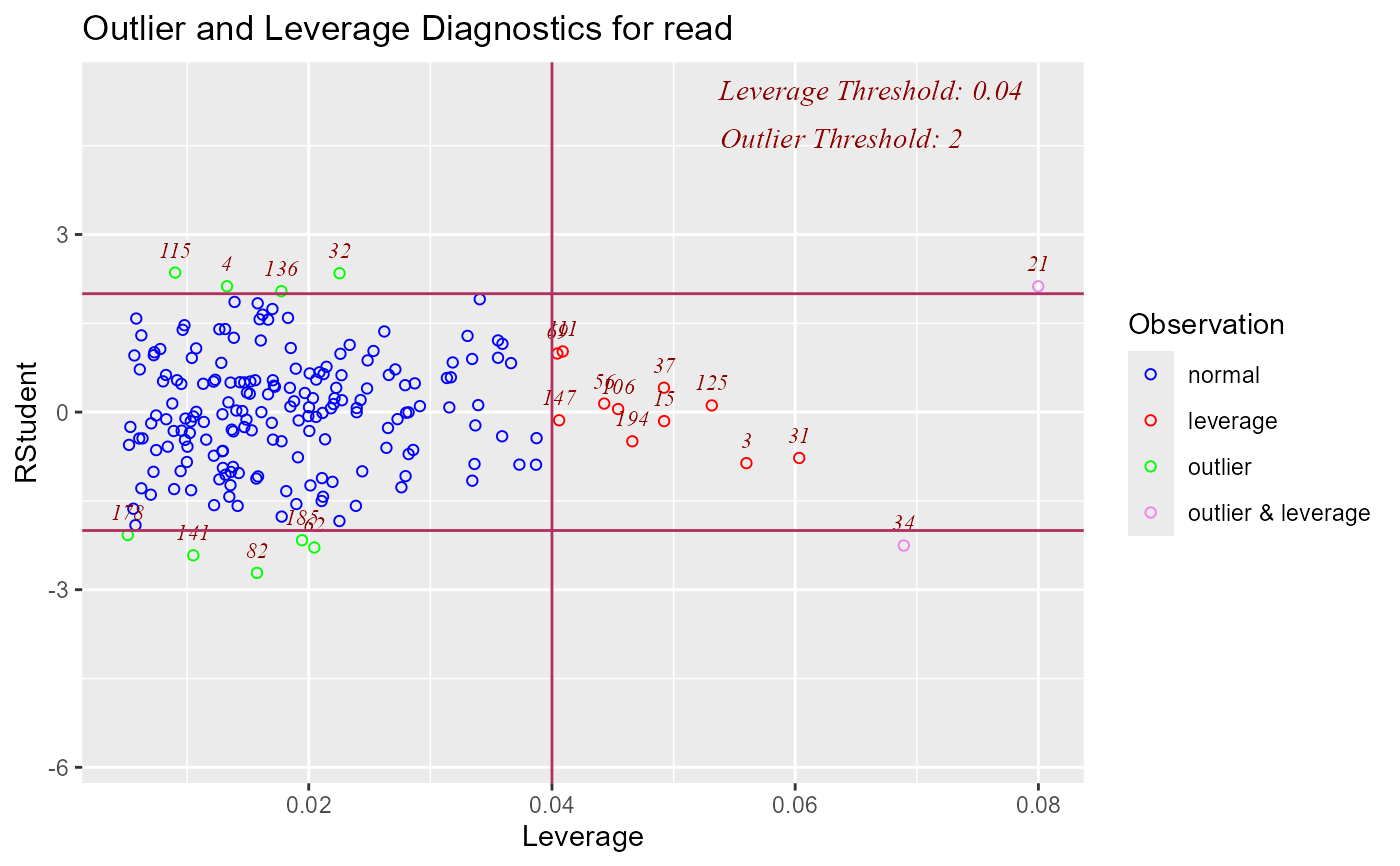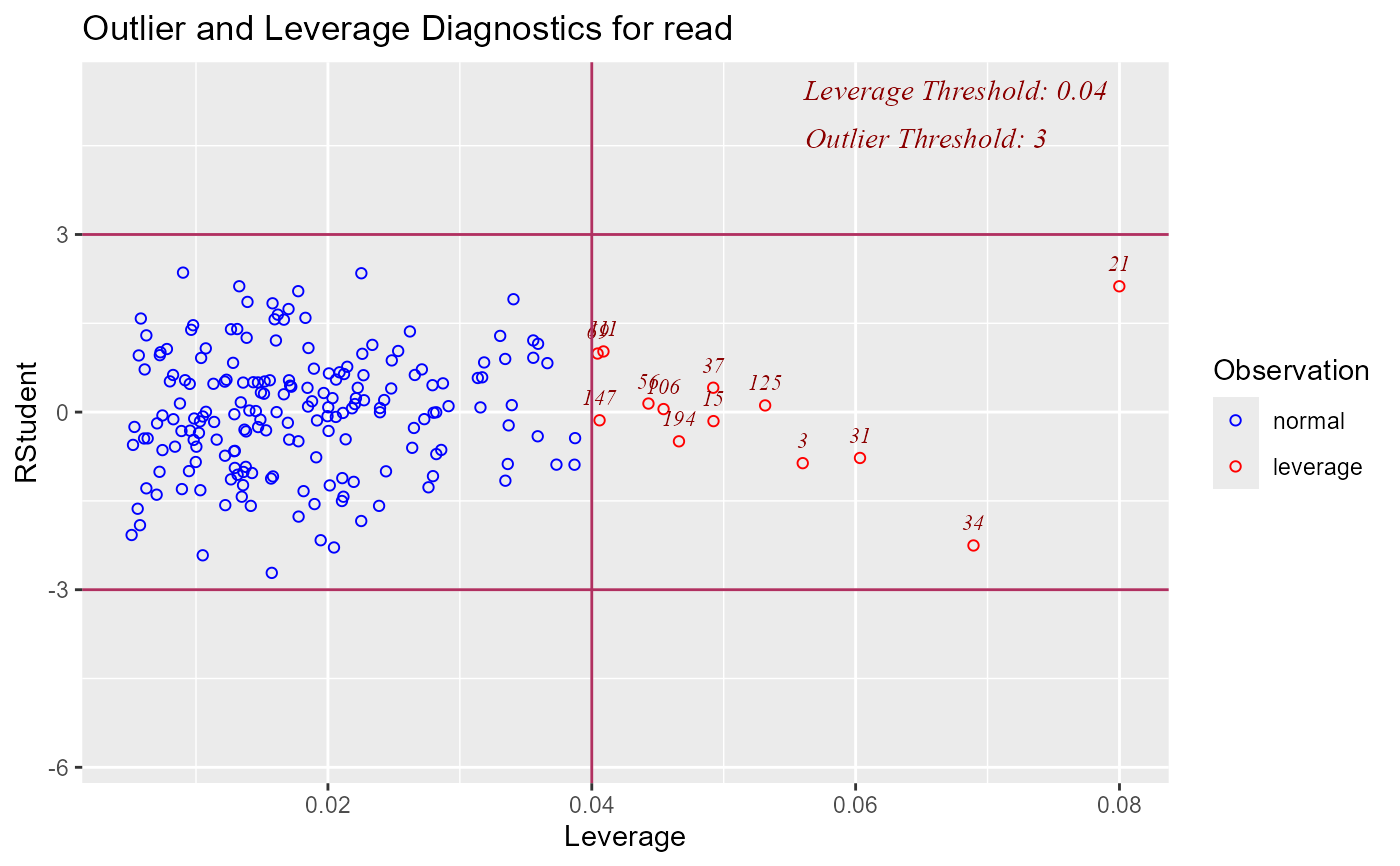Graph for detecting outliers and/or observations with high leverage.

ols_plot_resid_lev(model, threshold = NULL, print_plot = TRUE)

## Arguments

model An object of class lm. Threshold for detecting outliers. Default is 2. logical; if TRUE, prints the plot else returns a plot object.

## Deprecated Function

ols_rsdlev_plot() has been deprecated. Instead use ols_plot_resid_lev().

model <- lm(read ~ write + math + science, data = hsb)ols_plot_resid_lev(model, threshold = 3)